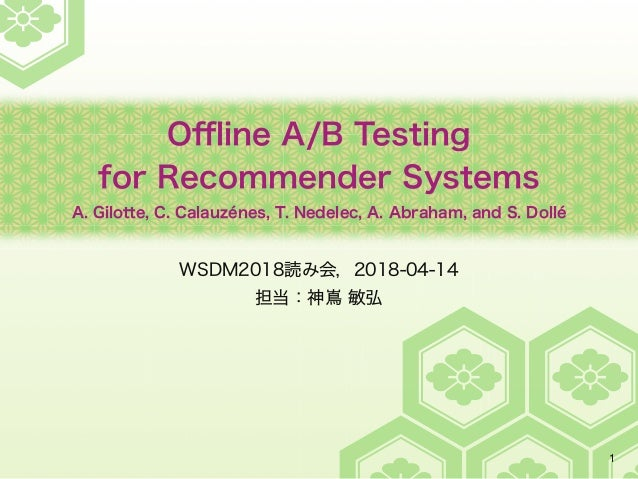Successfully reported this slideshow.Upcoming SlideShare
×

# WSDM2018読み会 資料

1,252 views

Published on

WSDM2018読み会
2018-04-14 @ クックパッド

https://atnd.org/events/95510

Offline A/B Testing for Recommender Systems
A. Gilotte, C. Calauzénes, T. Nedelec, A. Abraham, and S. Dollé

https://doi.org/10.1145/3159652.3159687

Published in: Data & Analytics
• Full Name
Comment goes here.

Are you sure you want to Yes No• Be the first to comment

• Be the first to like this

### WSDM2018読み会 資料

1. 1. Oﬄine A/B Testing for Recommender Systems A. Gilotte, C. Calauzénes, T. Nedelec, A. Abraham, and S. Dollé WSDM2018読み会，2018-04-14 担当：神嶌 敏弘 1
2. 2. 概要 2 オンラインでは，現状 prod（対照群）と施策 test（実験群）を実 際に行って比較する これを低コストな prod のデータだけで低コストで実施したい BIS：施策で生じる分布の差で重み付けした重点サンプリング 不偏推定量だが，バリアンスが大きすぎる CIS：施策の効果が大きすぎる部分の影響をカットして分散を抑制 バリアンスは大きく減少するが，バイアスが生じる NCIS：バイアスを大域的に推定して補正 バイアスはちょっと補正できた PieceNCIS，PointNCIS：施策への入力に応じて局所的に補正する 提案手法 バイアスはとても減った
3. 3. 準備 3 X：状況，特徴ベクトル，広告を出すサイトや閲覧者の特徴を示す A：行動 ̶ 推薦結果・表示する広告，定義域は大きい R：報酬，クリック率など 方策 πp (prod) と πt (test) は π(A | X) の確率分布 オンラインテスト：ΔR = Eπp[R] − Eπt[R] の効果を調べる 期待値はそれぞれの方策下で集めた標本から推定する オフラインテスト：方策 p の下での標本 Sp のみが与えられる ΔR̂ を推定するのに Eπt[R] をどうにか Sp から推定する必要 バイアスとバリアンス：いろいろな標本から推定した推定量の，母 集団の値に対する偏りとばらつき
4. 4. Basic Importance Sampling 4 πt や πp は，自分で設計したものだから計算できる バイアスは 0 の不偏推定量 方策 p と t の乖離が大きいとバリアンスは大きくなる バリアンスが大きいと，本当に p と t で差があるかどうか不明に → このバリアンスを小さくしたい ˆRIS (πt ) = 1 n X (x,a,r )2Sn w(a,x)r where w(a,x) = πt (a|x) πp (a|x) 報酬重み 標本の大きさ 方策 p での標本
5. 5. Doubly Robust Estimator 5 方策 t での報酬の良い推定量があるとき，観測値との差だけを重点 サンプリングすることでバリアンスを減らす 推薦の行動 A の定義域は大きいので，よい推定量を構成するのはほ ぼ無理 元々の R のバリアンスが大きいと，推定量との相関はどうやっても 小さい（Rがスパースな場合など） ˆRDR (πt ) = X (x,a,r )2Sn (r − ¯r (a,x)) w(a,x) + Eπt [¯r (A,X )|X = x] ! 方策 t での報酬の良い推定量
6. 6. Normalized Importance Sampling 6 重み W の方策 p での期待値は1だが，有限の大きさの標本ではそう はならない 不偏推定量にはならないが，バリアンスはBISより小さい 少なくとも漸近的には不偏性をあきらめないとバリアンスを小さく するのは無理 [ ] ˆRNIS (πt ) = 1 P (x,a,r )2Sn w(a,x) X (x,a,r )2Sn w(a,x)r n ではなく標本から求める
7. 7. Capped Importance Sampling 7 caping：しきい値 c と比較して大きすぎる重みを抑制 バリアンスは小さくなるが，バイアスを生じる cap した重みは以後 w̄(a, x) を記す ˆRmaxCIS (πt ,c) = 1 n X (x,a,r )2Sn min(w(a,x),c)r ˆRzeroCIS (πt ,c) = 1 n X (x,a,r )2Sn 1w(a,x)<cw(a,x)r 重みはたかだか c c 以上の重みは無視
8. 8. CISのバイアス 8 cap されるときの報酬の期待値が小さくないとバイアスは大きい → 方策 test で高頻度になるとき報酬は小さい → そんな方策は悪い方策… よいバイアス・バリアンスのバランスをとれる c は存在しない 図2：1%ほどの改善を検出しようとしたときの水準とくらべて， バイアス・バリアンスが共にこの水準を下回ることはない Eπt [R] = Eπp [ ˆRCIS (πt ,c)] | {z } RCIS (πt,c) + Eπt 266664 R W −W W ������ W > c 377775 Pπt (W > c) | {z } BCIS (πt,c)
9. 9. Normalized Capped Importance Sampling 9 NISとCISの組み合わせ capしていない部分の推定量を，capした部分に比例配分している 大域的なバイアスのモデルを使って，バイアスを補正したことに該 当 バリアンスをさらに小さくするには，入力 X に依存した補正が必要 （細かい議論は理解できなかった…） ˆRNCIS (πt ,c) = 1 n P (x,a,r )2Sn w(a,x)r 1 n P (x,a,r )2Sn w(a,x) Eπt  W R W � Eπt  W W � =漸近的にこの値になる →
10. 10. Piecewise NCIS 10 X をグループに分けて，それぞれで推定する うまいグループ分けを見つけなければならない x∈X の報酬値が似ているグループを見つけるとよい Eπt [R] = X д2G Eπt [R|X 2 д]P(X 2 д) X のグループ グループ内でNCIS
11. 11. Pointwise NCIS 11 さらに局所化して，x ごとに推定 ある x での報酬の期待値を計算するのは困難 E[W̄ /W | x] は，πt からのサンプリングで計算可能 Eπt [R] = X x 2X Eπt [R|X = x]P(X = x) X のそれぞれの値 同じ x のでの期待値 Eπt [R|X = x] ⇡ Eπt  RW W ���� X = x � Eπt  W W ���� X = x � ˆRPointNCIS (πt ,c) = 1 n X (x,a,r )2Sn ˆIPc (x)w(a,x)r
12. 12. 実験 12 39種類の数100G件のログ オンラインとオフラインのΔRの間の相関 CIS は逆相関と悪い バイアスが大域補正(NCIS)より局所補正(PieaceNCIS/PointNCIS) がよい
13. 13. 実験 13 ΔRの90%信頼区間，赤：偽負，橙：偽正，緑：正解 無効なものを運用に入れてしまう偽正の方が悪い 横軸はオフライン，縦軸はオンライン CISが真値と負相関になるのは，全般的に予測が低めであるため